# pandas数据分析之合并Excel文件Python算法之旅 |1122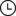2021-12-27 04:03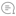0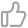00

pandas数据分析在教材中占比不高，估计考试中出现的概率也不会太大。但是pandas的功能实在太强大了，不用它来做点什么东西实在说不过去。

1展示了4Excel文件，第一份“总名单”记录了所有学生的姓名和班级信息，另外3份数据文件分别表示不同老师记录的学生成绩。其中“数据1”记录了2个班所有同学的数学成绩，“数据2”记录了1班的英语和2班的语文成绩，“数据3”记录了1班的语文和2班的英语成绩。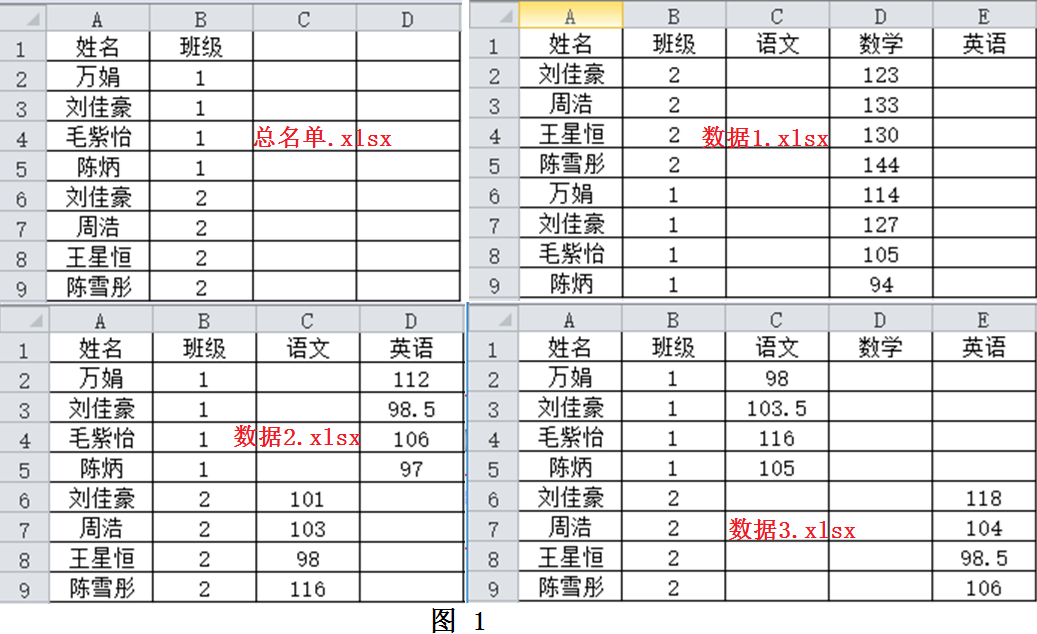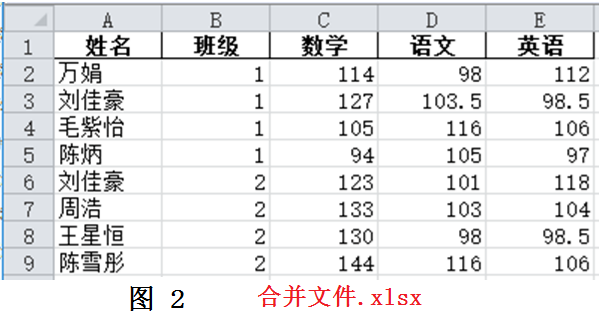pandas是基于numpy的数据分析模块，提供了大量标准数据模型和高效操作大型数据集所需的工具，能够快速便捷地进行数据分析和处理。我们今天就使用pandas模块来编程解决合并Excel文件的问题。

``importpandas as pd      #导入pandas模块``file_name= "总名单.xlsx"``df = pd.read_excel(file_name)``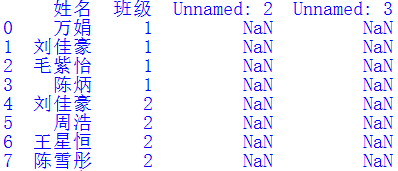``df =df.dropna(axis=1,how='all')  #清除df中一整列都是缺失值的列``#也可以写作：df.dropna(axis=1,how='all',inplace=True)``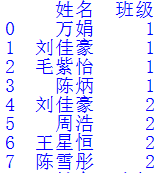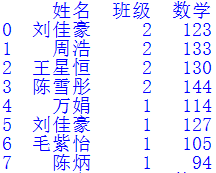``file_name = "数据1.xlsx"      ``df2 = pd.read_excel(file_name)``df2 = df2.dropna(axis=1, how='all')``cols = ["姓名","班级"]``df = pd.merge(df, df2, how='left', on=cols)``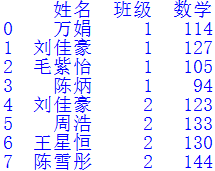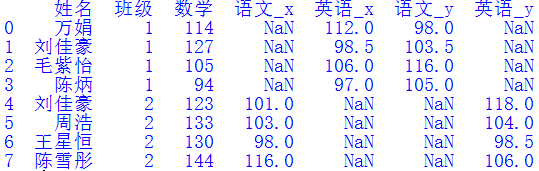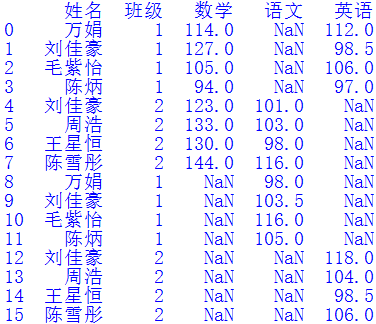`` df.fillna(df2, inplace=True)``

fillna()函数可以使用指定方法填充Dataframe对象的NaN值，在本例中df2中的语文和英语列恰好可以把原来df中的缺失值补上。问题完美解决。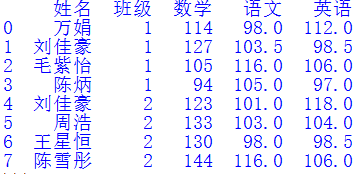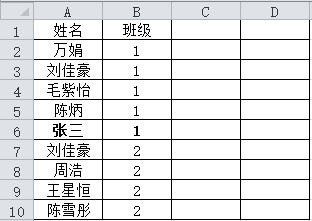``a.set_index(["班级","姓名"], inplace=True)``b.set_index(["班级","姓名"], inplace=True)``a.combine_first(b)``

set_index()函数我是知道的，因为它经常和join()函数一起使用；但combine_first()函数我确实是第一次见到。学艺不精啊！

``cols = ["姓名","班级"]``df.set_index(cols, inplace=True)``df2.set_index(cols, inplace=True)``df = df.combine_first(df2)````df.sort_values(by=["班级","姓名"], inplace=True)``df.reset_index(inplace=True)``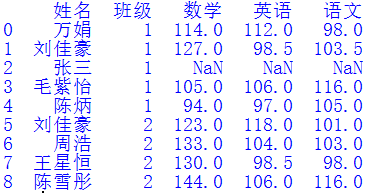``#!/usr/bin/python3``# 文件名: pandas应用之合并Excel文件``# 作者：巧若拙``# 时间：2021-12-25``import os, sys``import pandas as pd``pd.set_option('display.unicode.ambiguous_as_wide', True)``pd.set_option('display.unicode.east_asian_width', True) #中英文字符对齐``file_name = "总名单.xlsx"``df = pd.read_excel(file_name)``df = df.dropna(axis=1, how = 'all') #丢弃所有列中所有值均缺失的列``basic_cols = list(df.columns) #获取基本列``df.set_index(basic_cols, inplace=True)``path = "合并文件夹/"``for file in os.listdir(path):``    try:``        iffile.index(".xls") or file.index(".xlsx"):``            new_df =pd.read_excel(path+file)``            new_df =new_df.dropna(axis=1, how='all')``           new_df.set_index(basic_cols, inplace=True)``            df =df.combine_first(new_df)``    except ValueError:``            print(f"{file}不是需要的文件")``df.reset_index(inplace=True)``print(df)``writer_file_name = "合并文件.xlsx"``writer = pd.ExcelWriter(writer_file_name)``df.to_excel(writer, sheet_name='汇总表', index=False)``writer.save()``

pandas在金融、统计、社会科学、工程等领域都有着广泛的应用，它提供的大量功能函数可以帮助我们快速方便地进行数据整理、分析和统计工作。但武器再好，不会用也是白搭。要想熟练使用这把“好剑”，就必须进一步深入学习，阅读相关教程和文档，提高自己的工作效率和编程水平。00

暂无评论~~
Ctrl+Enter